• +91 9971497814
• info@interviewmaterial.com

# Chapter 5 Understanding Elementary Shape Ex-5.1 Interview Questions Answers

### Related Subjects

Question 1 :  What is the disadvantage in comparing line segments by mere observation?

Answer 1 : By mere observation we can’t compare the line segments with slight difference in their length. We can’t say which line segment is of greater length. Hence, the chances of errors due to improper viewing are more.

Question 2 : Why is it better to use a divider than a ruler, while measuring the length of a line segment?

Answer 2 : While using a ruler, chances of error occur due to thickness of the ruler and angular viewing. Hence, using divider accurate measurement is possible.

Question 3 : Draw any line segment, say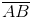. Take any point C lying in between A and B. Measure the lengths of AB, BC and AC. Is AB = AC + CB?

Since given that point C lie in between A and B. Hence, all points are lying on same line segment. Therefore for every situation in which point C is lying in between A and B we may say that
AB = AC + CB
For example:
AB is a line segment of length 7 cm and C is a point between A and B such that AC = 3 cm and CB = 4 cm.
Hence, AC + CB = 7 cm
Since, AB = 7 cm
∴ AB = AC + CB is verified.

Question 4 : If A, B, C are three points on a line such that AB =5 cm, BC = 3 cm and AC = 8 cm, which one of them lies between the other two?

Given AB = 5 cm
BC = 3 cm
AC = 8 cm
Now, it is clear that AC = AB + BC
Hence, point B lies between A and C.

Question 5 : Verify, whether D is the mid point of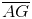.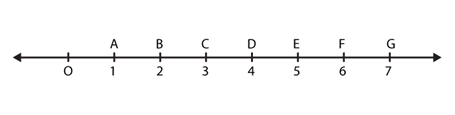Answer 5 : Since, it is clear from the figure that AD = DG = 3 units. Hence, D is the midpoint ofQuestion 6 : If B is the mid point of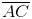and C is the mid point of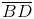, where A, B, C, D lie on a straight line, say why AB = CD?

Answer 6 :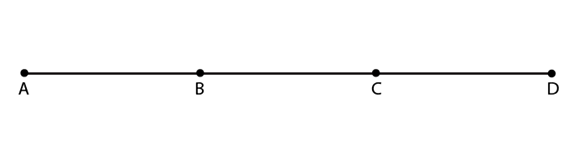Given
B is the midpoint of AC. Hence, AB = BC (1)
C is the midpoint of BD. Hence, BC = CD (2)
From (1) and (2)
AB = CD is verified

Question 7 : Draw five triangles and measure their sides. Check in each case, if the sum of the lengths of any two sides is always less than the third side.

Answer 7 : Case 1. In triangle ABC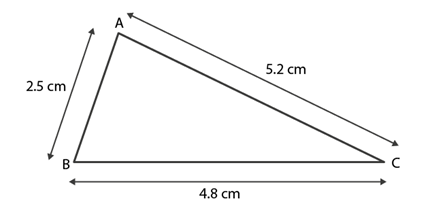AB= 2.5 cm
BC = 4.8 cm and
AC = 5.2 cm
AB + BC = 2.5 cm + 4.8 cm
= 7.3 cm
As 7.3 > 5.2
∴ AB + BC > AC
Hence, the sum of any two sides of a triangle is greater than the third side.
Case 2. In triangle PQR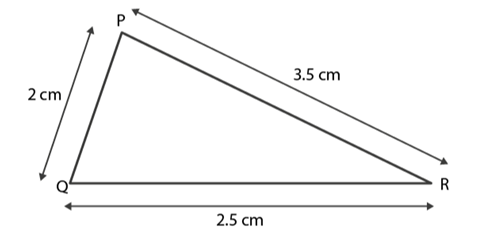PQ = 2 cm
QR = 2.5 cm
PR = 3.5 cm
PQ + QR = 2 cm + 2.5 cm
= 4.5 cm
As 4.5 > 3.5
∴ PQ + QR > PR
Hence, the sum of any two sides of a triangle is greater than the third side.
Case 3. In triangle XYZ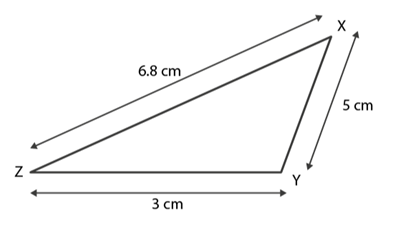XY = 5 cm
YZ = 3 cm
ZX = 6.8 cm
XY + YZ = 5 cm + 3 cm
= 8 cm
As 8 > 6.8
∴ XY + YZ > ZX
Hence, the sum of any two sides of a triangle is greater than the third side.
Case 4. In triangle MNS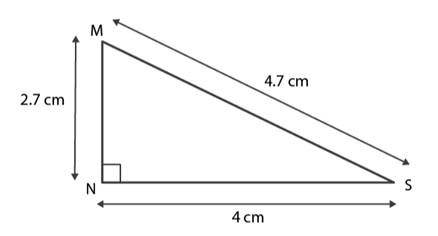MN = 2.7 cm
NS = 4 cm
MS = 4.7 cm
MN + NS = 2.7 cm + 4 cm
6.7 cm
As 6.7 > 4.7
∴ MN + NS > MS
Hence, the sum of any two sides of a triangle is greater than the third side.
Case 5. In triangle KLM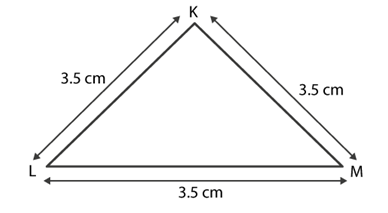KL = 3.5 cm
LM = 3.5 cm
KM = 3.5 cm
KL + LM = 3.5 cm + 3.5 cm
= 7 cm
As 7 cm > 3.5 cm
∴ KL + LM > KM
Hence, the sum of any two sides of a triangle is greater than the third side.
Therefore we conclude that the sum of any two sides of a triangle is always greater than the third side.

Todays Deals### Chapter 5 Understanding Elementary Shape Ex-5.1 Contributorskrishan

Name:
Email:

# Latest News# 9000 interview questions in different categories